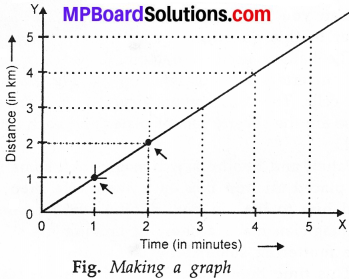## MP Board Class 7th Science Solutions Chapter 13 Motion and Time

### Motion and Time Intex Questions

Question 1.
Paheli wondered how time was measured when pendulum clocks were not available?
Many times measuring devices were used in different parts of the world before the pendulum clocks became popular. Water clocks, sand clocks and sundials are some examples of such devices.

Question 2.
Boojho wants to know whether there is any device that measures the speed?
Yes, speedometer.

Activities

Activity – 1
Table gives some common examples of motions. Identify the type of motion in each case?
Some examples of different types of motion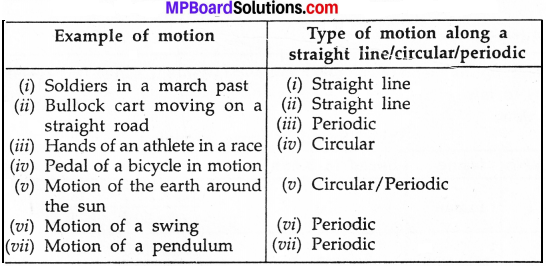Activity – 2
Draw a straight line on the ground with chalk powder or lime and ask one of your friends to stand 1 to 2 m away from it. Let your friend gently roll a ball along the ground in a direction perpendicular to the line. Note the time at the moment the ball crosses the line and also when it comes to rest (Fig.) How much time does the ball take to come to rest? Measure the distance between the point at which the ball crosses the line and the point where it comes to rest. You can use a scale or a measuring tape. Let different groups repeat the activity. Record the measurements in Table. In each case calculate the speed of the ball?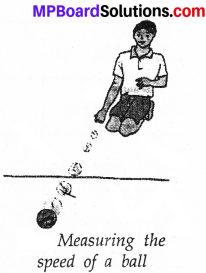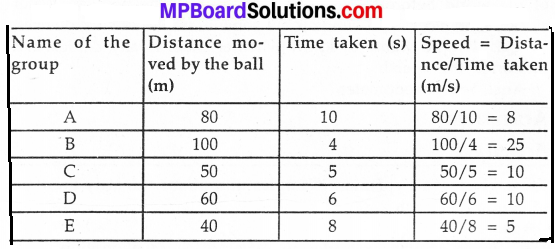Activity – 3
The unit of speed in the given table is in km/h. Change this speed in to m/s?
Speed of some animals.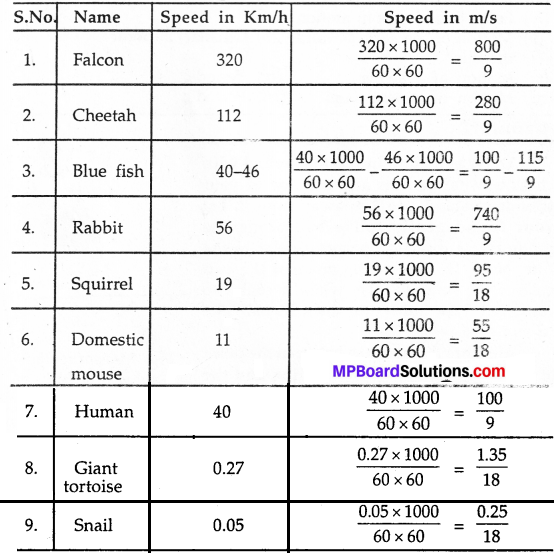### Motion and Time Text Book Exercises

Question 1.
Classify the following as motion along a straight line, circular or oscillatory motion:

1. Motion of your hands while running.
2. Motion of a horse pulling a cart on a straight road.
3. Motion of a child in a merry – go – round.
4. Motion of a child on a see – saw.
5. Motion of the hammer of an electric bell.
6. Motion of a train on a straight bridge.

1. Oscillatory
2. Straight line
3. Circular
4. Oscillatory
5. Oscillatory
6. Straight line.Question 2.
Which of the following are not correct?

1. The basic unit of time is second.
2. Every object moves with a constant speed.
3. Distances between two cities are measured in kilometres.
4. The time period of a given pendulum is not constant.
5. The speed of a train is expressed in m/h.

2. Every object moves with a constant speed.
5. The speed of a train is expressed in m/h.

Question 3.
A simple pendulum takes 32 s to complete 20 oscillations?What is the time period of the pendulum?
Given, time taken to complete 20 oscillations = 32 seconds
∴Time taken to complete 1 oscillation = $$\frac{32}{20}$$s = 1.6 s
Thus, the time period of pendulum is 1.6 s

Question 4.
The distance between two stations is 240 km. A train takes 4 hours to cover this distance. Calculate the speed of the train?
Given, distance between two stations = 240 km and time taken to cover this distance = 4 hour
We know that,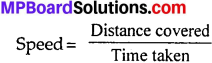$$\frac{240}{4}$$ = 60 km/hour.
Thus, the speed of the train is 60 km/hour.

Question 5.
The odometer of a car reads 57321.0 km when the clock shows the time 08.30 AM. What is the distance moved by the car, if at 08:50 AM, the odometer reading has changed to 57336.0 km? Calculate the speed of the car in km/min during this time. Express the speed in km/h also?
Given, reading of odometer at time 08:30 AM = 57321.0 km and reading of odometer at time 08:50 AM = 57336.0 km.
∴Distance covered by car = (57336.0 – 57321.0) km = 15 km and Time taken = 8.50 – 8.30 = 20 minutes
We know that,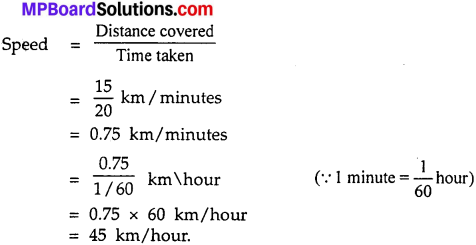Question 6.
The odometer of a car reads 57321.0 km when the clock shows the time 08.30 AM. What is the distance moved by the car, if at 08:50 AM, the odometer reading has changed to 57336.0 km? Calculate the speed of the car in km/min during this time. Express the speed in km/h also?
Given, reading of odometer at time 08:30 AM = 57321.0 km and reading of odometer at time 08:50 AM = 57336.0 km
∴Distance covered by car = (57336.0 – 57321.0) km = 15 km and Time taken = 8.50 – 8.30 = 20 minutes We know that,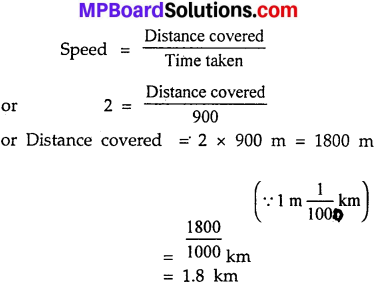Thus, the distance between Salma’s school from her house is 1800 m or 1.8 km.

Question 7.
Show the shape of the distance – time graph for the motion in the following cases:

1. A car moving with a constant speed.
2. A car parked on a side road.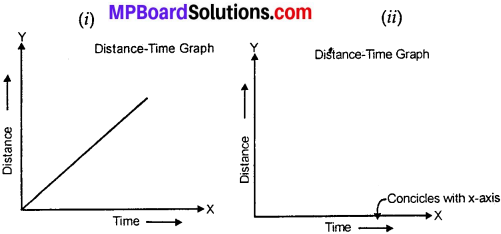Question 8.
Which of the following relations is correct?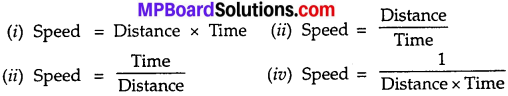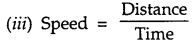Question 9.
The basic unit of speed is:
(a) 100km.
(b) m/min
(c) km/h
(d) m/s.
(d) m/s.

Question 10.
A car moves with a speed of 40 km/h for 15 minutes and then with a speed of 60 km/h for the next 15 minutes. The total distance covered by the car is:
(a) 100 km
(b) 25 km
(c) 15 km
(d) 10 km.
(b) 25 km.
Distance travelled in first 15 minutes = Speed x Time = 40 km/h x 15 minutes.
= 40 km/h × $$\frac{15}{60}$$ hour
= 10 km
Distance travelled in last 15 minutes = Speed x Time = 60 km/h x 15 minutes
= 60 km/h × 15 minutes
= 60 km/h × $$\frac{15}{60}$$ hour
= 15 km.
Total distance = (10 + 15) km = 25 km.

Question 11.
Suppose the two photographs, shown in Fig. (a) and Fig. (b) had been taken at an interval of 10 seconds. If a distance of 100 meters is shown by 1 cm in these photographs, calculate the speed of the blue car?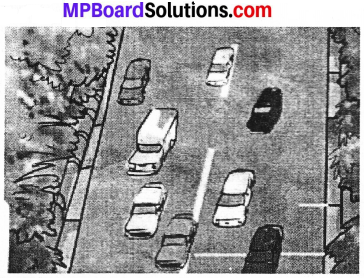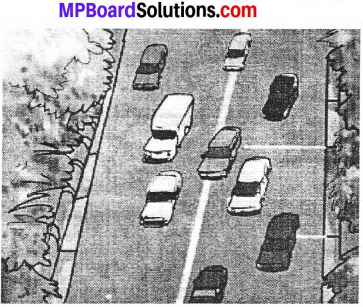Speed = $$\frac{100 km}{10 h}$$ = 10 km/h.

Question 12.
Fig. shows the distance – time graph for the motion of two vehicles A and B. Which one of them is moving faster?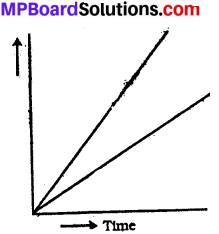Car A is moving faster.

Question 13.
Which of the following distance – time graphs shows a truck moving with speed which is not constant?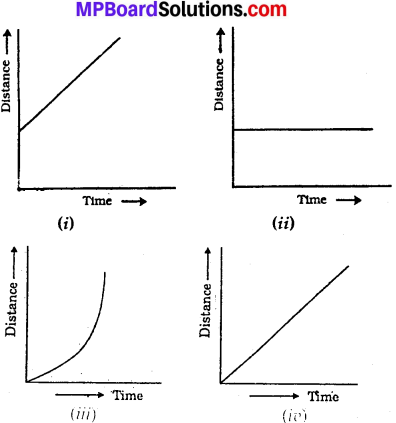(iii)

### Motion and Time Additional Important Questions

Objective Type Questions

Question 1.
Choose the correct alternative :

Question (a)
The time taken for one oscillation is called its
(a) Pitch
(b) Frequency
(c) Vibration
(d) Time period.
(d) Time period.

Question (b)
1 minute has?
(a) 30 second
(b) 60 second
(c) 100 second
(d) None of these.
(b) 60 secondQuestion (c)
The smallest time interval that can be measured with commonly available clocks and watches is?
(a) 1 second
(b) 1 minute
(c) 1 hour
(d) None of these.
(c) 1 hour

Question (d)
The ages of stars and planet are often expressed in –
(a) billions of years
(b) days
(c) years
(d) None of these.
(a) billions of years

Question (e)
The distance moved by a vehicle can be measured by –
(a) speedometer
(b) odometer
(c) regulator
(d) None of these.
(b) odometer

Question (f)
The distance – time graph of a body moving with uniform speed is –
(a) a curve
(b) a straight line
(c) line parallel to x – axis
(d) None of these.
(b) a straight lineQuestion 2.
Fill in the blanks:

1. We shall use the term speed for average ……………………….
2. An object moving along a straight line with a constant speed is said to be in …………………………
3. The to and fro motion of a simple pendulum is an example of a ……………….. or ……………………. motion.
4. The basic unit of time is a ……………………….
5. The basic unit of speed is ………………………..
6. Periodic events are used for the measurement of ……………………….
7. The distance – time graph for the motion of an object moving with a constant speed is a ……………………
8. The speedometer records the speed in ……………………..
9. Odometer is used to measure ………………………
10. The distance moved by an object is a unit time is called its ………………………….

1. Speed
2. Uniform speed
3. Periodic, oscillatory
4. Second
5. m/s
6. Time
7. Straight line
8. km/h
9. Distance
10. Speed.

Question 3.
Which of the following statements are true (T) or false(F).

1. The distance moved by objects in a given interval of time can help us to decide which one is faster or slower.
2. We shall not use the term speed for average speed.
3. The to and fro motion of a simple pendulum is an example of a periodic motion.
4. Janter Manter in Delhi and Jaipur have big Sundials.
5. In non – uniform motion, the speed of an object changes.
6. Clocks or watches are perhaps the most common time measuring devices.
7. Different units of time are used depending on the need.

1. True
2. False
3. True
4. True
5. True
6. True
7. True.Motion and Time Very Short Answer Type Questions

Question 1.
Define motion.
Motion is the change in position of a body with respect to line and its surroundings.

Question 2.
What type of motion do the vehicles on a straight road perform?
The vehicles perform rectilinear motion on the road.

Question 3.
What type of motion does the wheel of a bicycle perform?
The wheel of a bicycle perform rotatory or circular motion.

Question 4.
What is uniform motion?
Motion of a body along a straight line covering equal distances in equal intervals of time is called uniform motion.Question 5.
Give two example of non – uniform motion?

1. Motion of the train when its driver applies breaks.
2. Motion of the cricket ball when the bowler throws the ball and the ball is hit by the player.

Question 6.
Give two example of periodic motion?

1. Rotation of earth on its axis.
2. Moon moves around the earth.

Question 7.
What is oscillatory motion?
The to and fro motion is called periodic or an oscillatory motion.

Question 8.
An object is hung from a spring is pulled down and left? What type of motion does the object perform?
The object perform oscillatory motion which is also a periodic motion.

Question 9.
Write the formula for speed?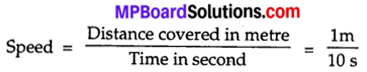Question 10.
What is the unit of speed?
Unit of speed is meter per second.

Question 11.
A snail covers a distance of 1 meter in 10 sec. What is the speed of the snail?
image
= 0.1 ms-1
= 0.1 m per sec.

Question 12.
What happens to the speed of your bicycle when you pedal faster?
On pedalling fast the speed of bicycle increase.

Question 13.
“Speed of a car is 8 km/h”. What does it mean?
When we say that a car is moving with a speed of 80 kilometer per hour, it implies that it will cover a distance of 80 kilometre in one hour.

Question 14.
Define a day?
The time between one sunrise and the next is called a day.

Question 15.
What is month ?
A month is measured from one new moon to the next.

Question 16.
What is year?
A year is fixed as the time taken by the earth to complete one revolution of the sun.

Question 17.
What is bob?
The metallic ball is called bob of the pendulum.Question 18.
What is the use of stop – watch?
Stop – watch is used to measure exact time in case of atheletic events as it can be stopped and started any moment.

Question 19.
When you travel in bus or car then, generally, what kind of motion it is?
Linear motion.

Question 20.
There is 100 metres line on which two sprinters have to run, when they run, what kind of motion it will be?
Linear motion.

Question 21.
See the picture and tell whose speed is more: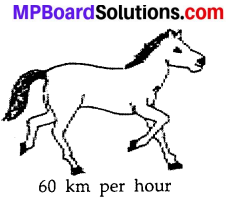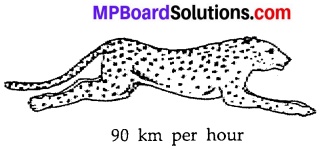Speed of tiger is more than the speed of horse.

Motion and Time Short Answer Type Questions

Question 1.
Define periodic motion?
Periodic motion:
The motion of a body which is repeated at regular intervals of time is called periodic motion. In periodic motion, a body covers equal distances in equal intervals of time; e.g. revolution of earth round the sun, the motion of moon round the earth, the motion of a swing, the motion of a pendulum, etc.

Question 2.
Define non – periodic motion?
Non – periodic motion:
The motion of a body which is not repeated at regular intervals of time is called non – periodic motion. In non – periodic motion, a body does not cover equal distances in equal intervals of time; e.g. an athelete running on a track, a moving car on busy road, etc.

Question 3.
Define oscillatory motion?
Oscillatory motion:
A body is said to be in oscillatory motion when it moves to and fro about a fixed point; e.g. the pendulum of a wall clock (Fig.), a swing, a wire of sitar when plucked, striking of drum, etc. Mostly the hanging objects show oscillatory motion.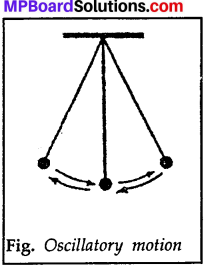Question 4.
How can we make a pendulum of air one? What is the time – period of a pendulum?
We can make a pendulum by suspending a metal ball with a cotton thread. The other end of the thread can be tied to same support. The time taken by a pendulum to complete its to and from movement i.e., one oscillation is called the time period of the pendulum.

Question 5.
One student reaches school in 1 hour by travelling a distance of 8 km from his house by a cycle and the other student reaches school in one and half hours by travelling a distance of 12 kms from his house by cycle. Tell whose speed was slow?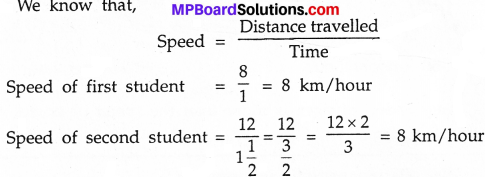From above calculations it is clear that the speed of both the students is same.

Question 6.
What are quartz clocks? Give a diagram of digital clock?
Now a days most clocks or watches have an electric circuit with one or more cells. These clocks are called quartz clocks. The time measured by quartz clocks is much more accurate than that by the clocks available earlier.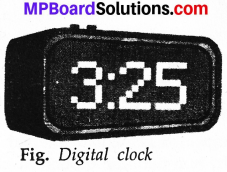Question 7.
Mark x – axis, y – axis, origin on a graph paper?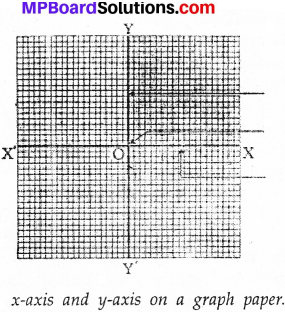Motion and Time Long Answer Type Questions

Question 1.
Write briefly about the development of pendulum clock?
There is an interesting story about the discovery that the time period of a given pendulum is constant. You might have heard the name of famous scientist Galileo Galilie (A.D. 1564 – 1642). It is said that once Galileo was sitting in a church. He noticed that a lamp suspended from the ceiling with a chain was moving slowly from one side to the other.

He was surprised to find that his pulse beat the same number of times during the interval in which the lamp completed one oscillation. Galileo experimented with various pendulums to verify his observation. He found that a pendulum of a given length takes always the same time to complete one oscillation. This observation led to the development of pendulum clocks. Winding clocks and wristwatches were refinements of the pendulum clocks.Question 2.
Discuss in details about the different range to measure time interval?
Or
Discuss the different units for measuring time interval?
The smallest time interval that can be measured with commonly available clocks and watches is one second. However, now special clocks are available that can measure time intervals smaller than a second. Some of these clocks can measure time intervals as small as one millionth or even one billionth of a second. We might have heard the terms like microsecond and nanosecond. One microsecond is one millionth of a second. A nanosecond is one billionth of a second.

Clocks that measure such small time intervals are used for scientific research. The time measuring devices used in sports can measure time intervals that are one tenth or one hundredth of a second. On the other hand, times of historical everts are stated in terms of centuries or millenniums. The ages of stars and planet are often expressed in billions of years.

Question 3.
Draw a bar graph to show the runs scored by a team in each over?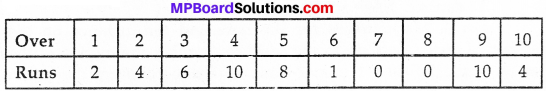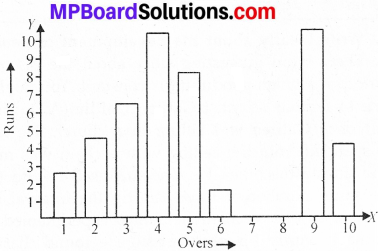Question 4.
Draw a pie chart to show the composition of air?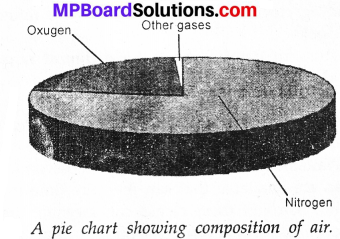Question 5.
Write down the steps to make a distance – time graph?
We can make the graph by following steps:
Step 1.
Draw two perpendicular lines to represent the two axes and mark them as OX and OY as shown in figure.

Steps 2.
Decide the quantity to be shown along the x – axis and that to be shown along the y – axis. In this case we show the time along the x – axis and the distance along the y – axis.

Steps 3.
Choose a scale to represent the distance and another to represent the time on the graph. For the motion of the car scales could be
Time : 1 min = 1 cm
Distance : 1 km = 1 cm

Step 4.
Mark values for the time and the distance on the respective axes according to the scale chosen. For the motion of the cat let mark the time 1 min, 2 min,..,on the origin x – axis from the origin Similarly, mark the distance 1 km. 2 km… on the y – axis.

Steps 5.
Now you have to mark the points on the graph paper to represent each set of values for distance and time.

Step 6.
Join all the points on the graph as shown in figure. It is a straight line. This is the distance-time graph for the motion of the car.
Note:
If the distance – time graph is a straight line, it indicates that the object is moving with a constant speed. However, if the speed of the object keeps changing, the graph can be of any shape.